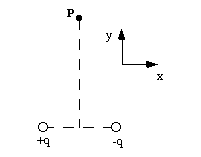### Physics 1B Thermal Physics & Electromagnetism

`H. E. Smith                                                                                                       Fall 2002`Physics 1B - Reading Quiz #41. In the hydrogen atom we find that the electrical force between the electron and proton is almost 1040 times greater than the gravitational force. Is there a finite distance between the particles such that the electrical force equals the gravitational force?
• A. Yes, if we move them much closer together.
• B. Yes, if we move them much farther apart.
• C. No, because the electrical and gravitational forces have the same distance dependence.

2. An electric dipole is placed at +/- x0 on the x-axis as shown below. What will be the direction of the resultant electric field at point P on the y-axis?
• A. in the +x direction (parallel to the x-axis).
• B. in the +y direction.
• C. in the -x direction.
• D. in another direction.
• E. The E-field at P is zero.